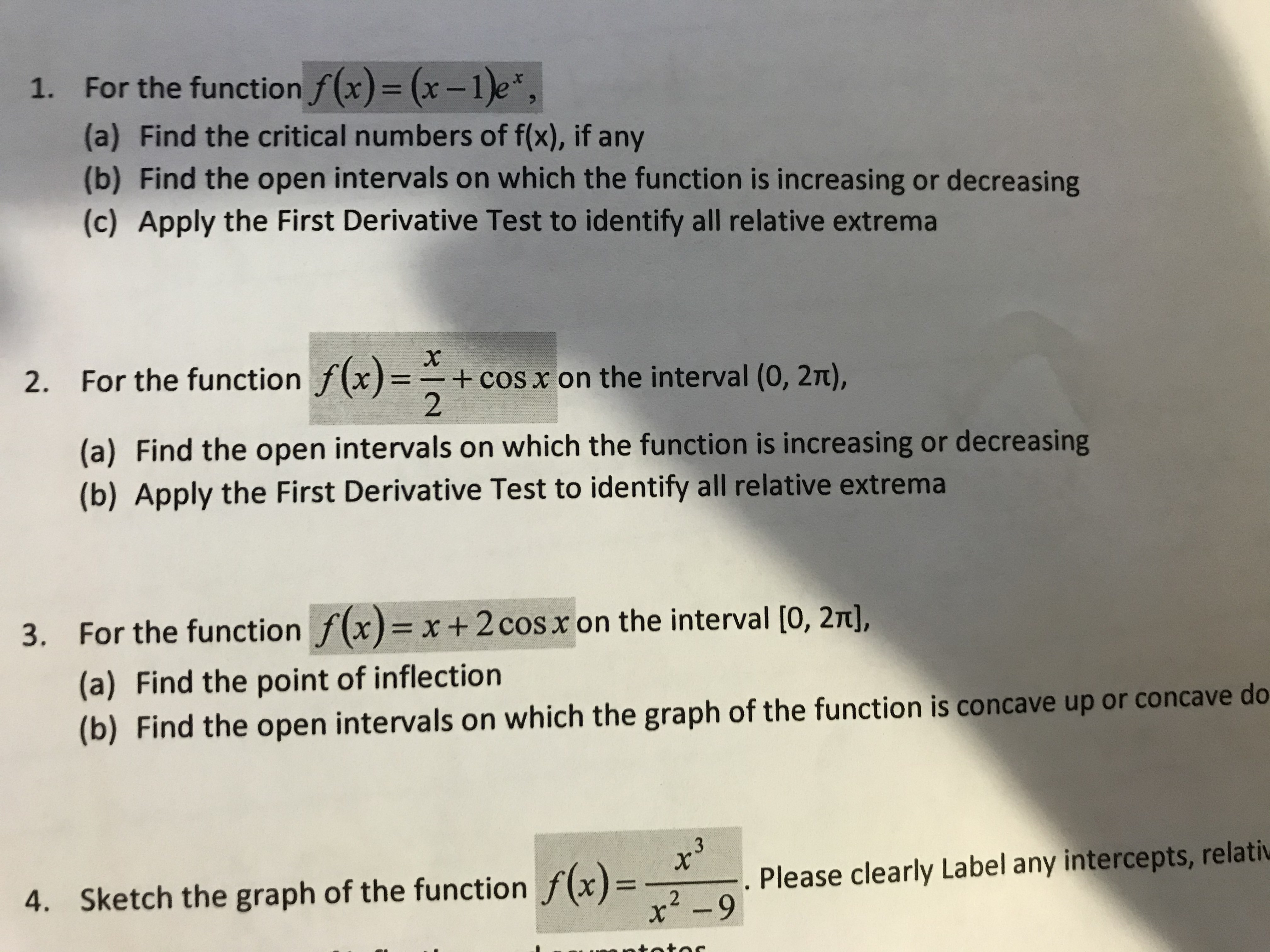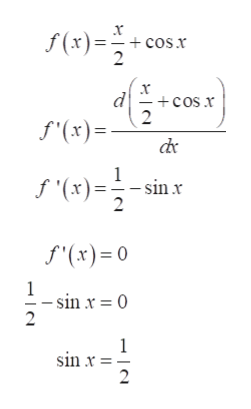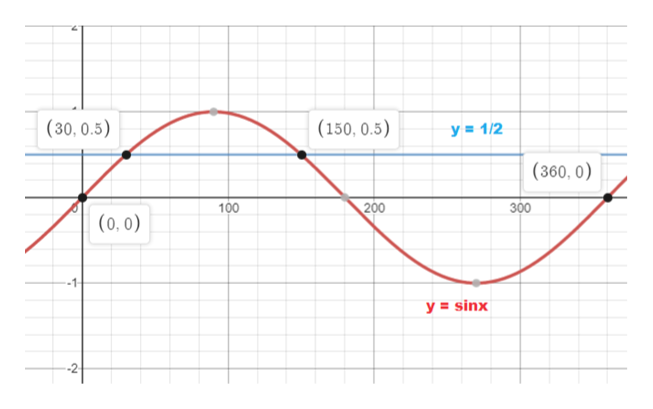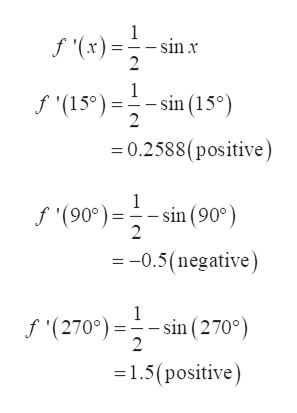# 1.For the function f(x) = (x-1)e,(a) Find the critical numbers of f(x), if any(b) Find the open intervals on which the function is increasing or decreasing(c) Apply the First Derivative Test to identify all relative extrema2. For the function f(x)=+ cos x on the interval (0, 2t),2(a) Find the open intervals on which the function is increasing or decreasing(b) Apply the First Derivative Test to identify all relative extrema3. For the function f(x) x+ 2 cos x on the interval [0, 2T],(a) Find the point of inflection(b) Find the open intervals on which the graph of the function is concave up or concave do3Sketch the graph of the function f(x)Please clearly Label any intercepts, relativ4.x2-9

Question
29 views

full workshelp_outlineImage Transcriptionclose1. For the function f(x) = (x-1)e, (a) Find the critical numbers of f(x), if any (b) Find the open intervals on which the function is increasing or decreasing (c) Apply the First Derivative Test to identify all relative extrema 2. For the function f(x)= + cos x on the interval (0, 2t), 2 (a) Find the open intervals on which the function is increasing or decreasing (b) Apply the First Derivative Test to identify all relative extrema 3. For the function f(x) x+ 2 cos x on the interval [0, 2T], (a) Find the point of inflection (b) Find the open intervals on which the graph of the function is concave up or concave do 3 Sketch the graph of the function f(x) Please clearly Label any intercepts, relativ 4. x2-9 fullscreen
check_circle

Step 1

(a) To find the function is increasing or decreasing, we need to know the critical points of the function in the given interval (0,2?).

To find the critical points, calculate f '(x) and equate it to 0.help_outlineImage Transcriptionclosef(x)= cos.x 2 x -cos r 2 d f'(x)= 1 sin x 2 f (x) f'(x)= 0 1 - sin x = 0 2 1 sinx - 2 fullscreen
Step 2

Now, value sin x is ½ in open interval (0,2?) at x = 30° and 150°help_outlineImage Transcriptionclose(30, 0.5) (150, 0.5) y 1/2 (360, 0) 200 300 100 (0,0) -1- y sinx -2- fullscreen
Step 3

Then, find the sign of f '(x) in the interval (0,30°), (30°,150°) and (150°,360°).

If f '(x) is positive, then f(x) is increasing in that interval and if it is negative then f(x) will be decreasing.

Moreover, if f '(x) = 0 then point of maxima and minima exists at that point.

Finding the value of f '(x) in x ∈  (0,30°...help_outlineImage Transcriptionclose1 - sin (x) 2 1 - sin (15°) 2 f(15)sin (15) =0.2588(positive) f (90)sin (90) 2 =-0.5(negative) 1 f '(270°)in(270°) 2 =1.5(positive) fullscreen

### Want to see the full answer?

See Solution

#### Want to see this answer and more?

Solutions are written by subject experts who are available 24/7. Questions are typically answered within 1 hour.*

See Solution
*Response times may vary by subject and question.
Tagged in
MathCalculus

### Functions